aCute A premium quality meshing software.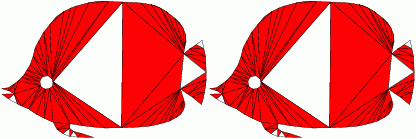Implemented and licensed at the University of Florida, Gainesville
Partially funded by an NSF CAREER Award and an NSF Regular Grant
Contact: Alper Ungor, CISE Department, University of Florida, Gainesville, FL, 32611 ungoratcisedotufldotedu

# Algorithmic Approach Comparison

#### Triangle

Our software employs an advancing front approach, inserts locally optimal Steiner points, first near the boundary of the domain.

The original Triangle software employed Ruppert's circumcenter insertion algorithm which is not an advancing front approach.

Below you see an execution of each algorithm/software interrupted after certain number of Steiner point insertion (specified). Desired smallest angle is 34 degrees. Bad triangles are marked red. Initial triangulations are the same.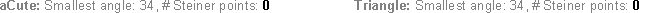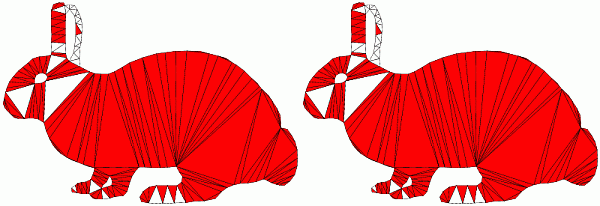# The Idea

Here, a brief discussion about the idea which helps us to generate premium quality triangulations by using Delaunay refinement as the basis

.

### Locally Optimal Steiner Points

Given an ordered pair of points (p,q) in the input domain, consider the circle that goes through p and q, of radius |pq| / (2*sin(alpha)), whose center is on the right side of the directed edge pq. The disk bounded by this circle is called the petal of pq, denoted by petal(pq). Based on this definition, we choose from four different types of points to insert a Steiner point which can be simply shown as follows:Find a point x inside the (shaded disk) petal of pq that is furthest away from all existing vertices. Such a point can be on a Voronoi edge (top) or a Voronoi vertex (bottom). Type I: on the dual of pq. Type II: on a Voronoi edge other than the dual of pq. Type III: a circumcenter other than that of pqr. Type IV: the circumcenter of pqr. Note that our approach nicely unifies the previously known Steiner point insertion techniques: circumcenter (Type V) of Chew and Ruppert, off-center (Type I) of Ungor, and sink (Type III) of Edelsbrunner and Guoy together with a new one (Type II).

Unlike the previous Delaunay refinement methods, we use circumcenters less frequently. This allows us to reach better minimum angle quality.

### Algorithm

 Compute the Delaunay triangulation of the input while there exists a bad triangle pqr with shortest edge pq       insert a point x is in petal(pq) of its shortest edge pq          that is furthest from all existing vertices

### Refinement and Relocation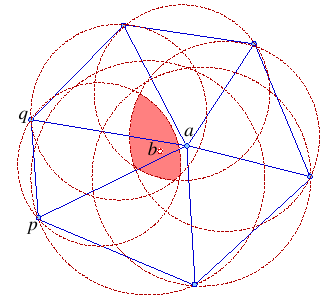Relocating a free vertex of a bad triangle pqr to the intersection of the petals of the link of a = r.

### Algorithm

 Compute the Delaunay triangulation (DelTri) of the input Let P denote the maintained point set while there exists a bad triangle pqr in DelTri(P) with shortest edge pq       relocated := False       for each free vertex a of pqr           if K = intersection of petal(xy), where xy is in link(a), is not empty set            and there exists a b in K such that all triangles of star(b) in the DelTri(P+{b}-{a}) are good           then               delete a; insert b; relocated:=True; break;       endfor       if relocated == False then           insert a point x is in petal(pq) of the bad triangle's shortest edge pq             that is furthest from all existing vertices endwhile

### Triangulations without Small and Large Angles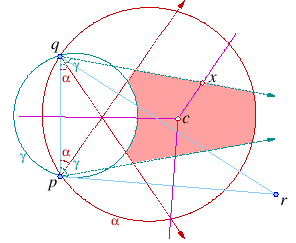Feasible region slice is the intersection of [alpha, gamma]-crescent, alpha- wedge, and gamma-slab. A point x inside this region that is furthest away from all existing vertices is chosen as a refinement point (left). A bad triangle pqa is fixed by relocating one of its vertices a into b which is a point in the intersection (shaded) of the [alpha, gamma]-slices of the edges on the link of a (right).

### Algorithm

 Compute the Delaunay triangulation (DelTri) of the input Let P denote the maintained point set while there exists a bad triangle pqr in DelTri(P) with shortest edge pq       relocated := False       for each free vertex a of pqr          forevery edge xy on the link of a            Compute alpha-wedge(xy)            Compute gamma-slab(xy)            Compute [alpha, gamma]-crescent(xy)            Compute [alpha, gamma]-slice(xy)         endfor         if K = intersection of [alpha, gamma]-slice(xy), where xy is in link(a), is not empty set            and there exists a b in K such that all triangles of star(b) in the DelTri(P+{b}-{a}) are good         then             delete a; insert b; relocated:=True; break;       endfor       if relocated == False then           insert a point x is in [alpha, gamma]-slice(xy) of the bad triangle's shortest edge pq             that is furthest from all existing vertices endwhile